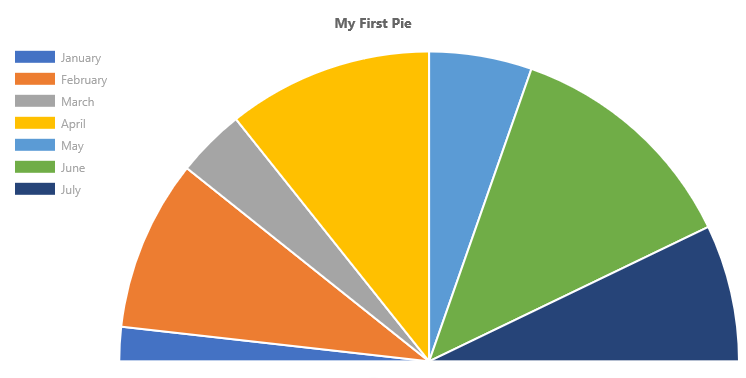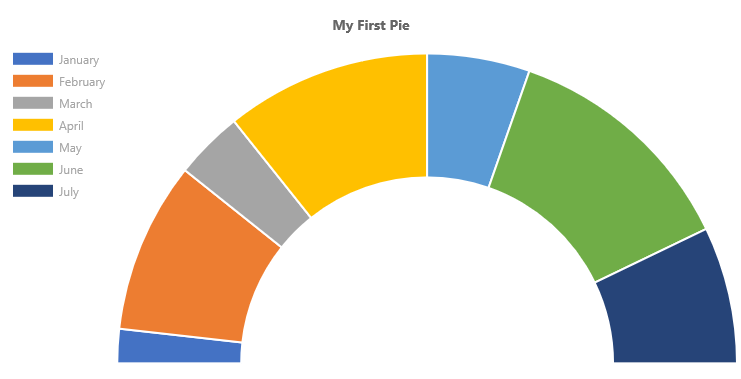# ChartControl - Pie Chart¶

Pie charts are divided into segments, each of which shows the proportional value of the data.## Example Usage¶

To create a pie chart, add the ChartControl import:

``````import { ChartControl, ChartType } from '@pnp/spfx-controls-react/lib/ChartControl';
``````

Then render the ChartControl:

`````` <ChartControl
type={ChartType.Pie}
data={data}
options={options}
/>
``````

For example, to render the chart above, use the following code:

``````// set the data
const data: Chart.ChartData = {
labels:
[
'January', 'February', 'March', 'April', 'May', 'June', 'July'
],
datasets: [
{
label: 'My First Dataset',
data:
[
10, 50, 20, 60, 30, 70, 40
]
}
]
};

// set the options
const options: Chart.ChartOptions = {
legend: {
display: true,
position: "left"
},
title: {
display: true,
text: "My First Pie"
}
};

return (
<ChartControl
type={ChartType.Pie}
data={data}
options={options}
/>);
``````

## Variations¶

### Half-Moon Pie charts¶By default, pie charts (and doughnut charts) render a whole circle. You can change the chart's `circumference` option to render partial circles.

The default `circumference` value is `2 * Math.PI`. To render a half-moon, specify a half value (i.e.: `Math.PI`), as follows:

`````` const options: Chart.ChartOptions = {
circumference: Math.PI,
legend: {
display: true,
position: "left"
},
title: {
display: true,
text: "My First Pie"
}
};
``````

Which renders the following half-moon:To rotate the pie chart 90 degrees to the left, specify a `rotation` value in the chart's options. For example, to render the horizontal half-moon chart shown at the top of this section, use the following options:

`````` const options: Chart.ChartOptions = {
circumference: Math.PI,
rotation: -1 * Math.PI,
legend: {
display: true,
position: "left"
},
title: {
display: true,
text: "My First Pie"
}
};
``````

### Doughnut charts¶

Technically, doughnut charts and pie charts are derived from the same class in Chart.js, where a doughnut chart's `cutoutPercentage` is set to 50.

If you wish to render simple doughnut charts, use the Doughnut Chart type.

However, if you wish to customize how the pie/doughtnut chart is rendered, you can set the cutout percentage to a different value.

For example, you can use the following code to render a custom "fuel-gauge" chart:

`````` const options: Chart.ChartOptions = {
circumference: 1 * Math.PI,
rotation: 1 * Math.PI,
cutoutPercentage: 60,
legend: {
display: true,
position: "left"
},
title: {
display: true,
text: "My First Pie"
}
};
``````

Will produce the following chart:## Dataset Properties¶

Pie charts allow each dataset to have different configuration properties.

Properties are provided as arrays. Settings in the array will be applied to each data element in the same order (e.g.: first value applies to first element, second value to second element, etc.)

Name Type Description
backgroundColor Color[] The segment's fill color.
borderColor Color[] The segment's border color.
borderWidth number[] The segment's border width. Measured in pixels.
data number[] The chart's data. Required.
hoverBackgroundColor Color[] The segment's fill color when a mouse hovers over it
hoverBorderColor Color[] The segment's border color when a mouse hovers over it.
hoverBorderWidth number[] The segment's border width when a mouse hovers over it.

## Data Structure¶

The `data` property of each dataset item consists of an array of numbers. Each point in the array corresponds to the matching label on the x axis:

``````data: [20, 10, 33, 47]
``````

## Configuration¶

The following configuration options are specific to pie charts:

Name Type Default Description
cutoutPercentage number 50 The percentage of the chart that is cut out of the middle.
rotation number -0.5 * Math.PI The angle at which the pie segments start
circumference number 2 * Math.PI The total circumference of the donut chart.
animation.animateRotate boolean true `true` will animate the chart while rotating it.
animation.animateScale boolean false `true` will animate the chart while scaling it.